#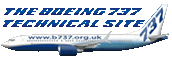737-300 V Speed Calculator

## Contents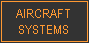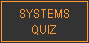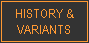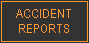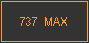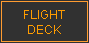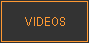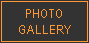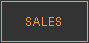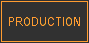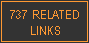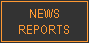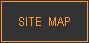### Contents

The following article, submitted by Captain Joseph Markham, provides pilots with a method of estimating V speeds for the 737-300 from the take-off weight. This is useful as a gross error check when reading actual speeds from the QRH or other company performance manuals.

All of the information, photographs & schematics from this website and much more is now available in a 374 page printed book or in electronic format.

*** Updated 23 Nov 2020 ***

 I’ve devised a V-speed calculator which I think is simple to use... Here goes….To calculate V2 first:…….. V-Speeds Flaps 1:   60 tons             V2=160             ( add 100 to the weight ) 50 tons             V2=145             (  -15  ) 40 tons             V2=130             (  -15 again ) You only have to remember this little table which, for 60t is 160, then subtract 15 for 50t and another 15 for 40t !!! For any weight in between, you simply interpolate… For Flaps 5, you subtract 5 from the speeds above, i.e Flap 5, 50t, V2 would be  145-5 = 140 etc For Flaps 15, subtract 15 from the speeds above, i.e. Flap 15, 50t, V2 would be 145-15 = 130 etc Now to calculate Vref:…….. V Refs Now, V2 for flaps 1 is vref for flaps 15, i.e  Flaps 15, 50t, Vref would be 145 For flaps 30, subtract 10 from the speed above, i.e. Flaps 30, 50t, Vref would be 145-10=135 For flaps 40, subtract 15 from the speed above, i.e. Flaps 40, 50t, Vref would be 145-15=130     The speeds are on the conservative side by a knot or so, but seem to work!!!!